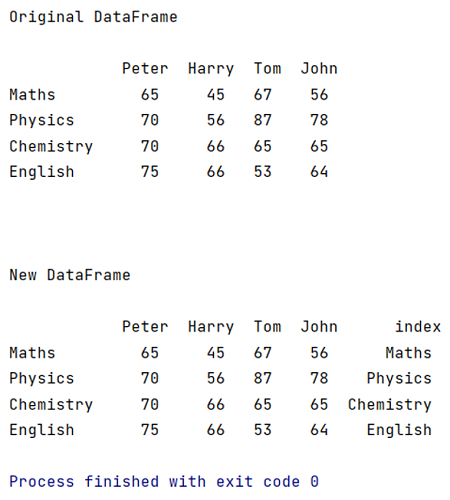# How to Convert Index to Column in Pandas Dataframe?

Learn how to convert index to column in Pandas Dataframe?
Submitted by Pranit Sharma, on April 13, 2022

Pandas is a special tool that allows us to perform complex manipulations of data effectively and efficiently. In a DataFrame, each row is assigned with an index value ranging from 0 to n-1. The 0th is the first row and n-1th index is the last row. Pandas provides us the simplest way to convert the index into a column.

We will create a new column and assign the index value of each row with the help of using DataFrame.index() method.

## pandas.DataFrame.index() Method

In Pandas DataFrame, both rows and columns have indexes, where each index value means that particular number of rows in a DataFrame. This method returns a list of all the index values which are assigned with each row.

Syntax:

```DataFrame.index()
```

To work with Python Pandas, we need to import the pandas library. Below is the syntax,

```import pandas as pd
```

Example:

```# Importing pandas package
import pandas as pd

# Creating a dictionary of student marks
d = {
"Peter":[65,70,70,75],
"Harry":[45,56,66,66],
"Tom":[67,87,65,53],
"John":[56,78,65,64]
}

# as subject names
df = pd.DataFrame(d,index=["Maths","Physics","Chemistry","English"])

# Printing the DataFrame
print("\noriginal DataFrame\n\n",df,"\n\n")

# Create a new column and insert its values
# using DataFrame.index() method
df['index'] = df.index

# Printing new DataFrame
print("\nNew DataFrame\n\n",df)
```

Output:

```
original DataFrame

Peter  Harry  Tom  John
Maths         65     45   67    56
Physics       70     56   87    78
Chemistry     70     66   65    65
English       75     66   53    64

New DataFrame

Peter  Harry  Tom  John      index
Maths         65     45   67    56      Maths
Physics       70     56   87    78    Physics
Chemistry     70     66   65    65  Chemistry
English       75     66   53    64    English
```What's New (MCQs)

Top Interview Coding Problems/Challenges!

IncludeHelp's Blogs

Languages: » C » C++ » C++ STL » Java » Data Structure » C#.Net » Android » Kotlin » SQL
Web Technologies: » PHP » Python » JavaScript » CSS » Ajax » Node.js » Web programming/HTML
Solved programs: » C » C++ » DS » Java » C#
Aptitude que. & ans.: » C » C++ » Java » DBMS
Interview que. & ans.: » C » Embedded C » Java » SEO » HR
CS Subjects: » CS Basics » O.S. » Networks » DBMS » Embedded Systems » Cloud Computing
» Machine learning » CS Organizations » Linux » DOS
More: » Articles » Puzzles » News/Updates